American Journal of Electrical Power and Energy Systems
Volume 5, Issue 5, September 2016, Pages: 45-50

Investigation & Minimization of Loss in Distribution System

Yalisho Girma Loaena

Department of Electrical & Computer Engineering, ArbaMinch University, ArbaMinch, Ethiopia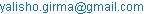Yalisho Girma Loaena. Investigation & Minimization of Loss in Distribution System. American Journal of Electrical Power and Energy Systems. Vol. 5, No. 5, 2016, pp. 45-50. doi: 10.11648/j.epes.20160505.11

Received: July 28, 2016; Accepted: September 5, 2016; Published: October 14, 2016

Abstract: For efficient and reliable operation of distribution system, the reactive power and voltage should be controlled so that energy loss should be minimized, bus-bar voltage should be maintained at acceptable limit (0.95P.U min and 1.05P.U max) and three phase voltages should approach as close as to the balanced system voltage . This paper work presents study of distribution system loss in the case of Textile factory, ArbaMinch town. The study makes a detail work on power system issues such as energy loss in the distribution system and its reduction techniques, reactive power flow and its compensation, power factor and voltage quality indicators such as voltage unbalance and voltage regulation. To achieve the objective, the existing distribution system of study site is modeled by using DIgSILENT Power Factory Software and simulation is carried out for unbalanced and balanced operation conditions. Clamp-on meter measurement is used to determine the load demand and line to line voltages. The results obtained for loss, power factor, voltage regulation and voltage unbalance are compared against accepted standards of IEEE, NEC & Ethiopian Electric Power Corporation (EEPCo) and possible solutions are recommended based on results obtained.

Keywords: Reactive Power, Energy Loss, Voltage Quality, Distribution System

Contents

1. Introduction

Distribution system is a part of electric power system that routes electric power to consumer loads with inductive, capacitive and resistive characteristics. It consists of network components such as distribution substation, primary and secondary distribution lines, distribution transformers, voltage regulators, shunt compensators and consumer loads. For efficient and reliable operation of distribution system, reactive power and voltage should be controlled so that overall power loss in the system is reduced to minimum value, voltage at load bus bars is maintained within acceptable NEC standard (0.95 to 1.05p.u) and three phase system voltages should approach near a balanced condition. Reactive power flow in distribution system is caused by a number of factors such as:

Line inductances

Transformer magnetization

Supplying reactive power requirement of the network components only by generating unit or substation transformer causes large system current and hence increases the power loss and voltage drop. In three phase system, the total reactive power is given by:

Q = √3 UL IL sin θ              (1)

Where, UL& IL are line voltage and line current respectively and θ is power factor angle. Reactive power in distribution system can be supplied by one of the following equipment. 

Synchronous condenser

Shunt capacitors

Power electronic based compensators (SVC)

The magnitude of the reactive power compensating devices can be determined by:

Q = P (tanθ1-tanθ2)             (2)

Where, "P" is active power demand, θ1 2 are power factor angles of original and compensated distribution system.

Based on the KVAr rating of the compensator, capacitance value is calculated as follows:

C = QC / V2ω               (3)

In three phase system where capacitors can be connected in either delta or star, it is convenient to use the per phase values.

For delta connected shunt capacitors, the per-phase capacitance is given by the following equation :

CΔ=QC/Vp2=QC/VL2ω            (4)

For star connected shunt capacitors, the per-phase capacitance is given as follows:

CY=QC/Vp2ω=3QC/VL2ω          (5)

The configuration whether delta or star depends on the voltage rating and capacitance of capacitors making up the bank.

The power factor angles of the distribution system can be determined by energy meter recordings as follows: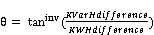(6)

Where KVArH difference & KWH difference are obtained by subtracting previously recorded value from its immediate next recorded value (time interval selected is usually 30 min or one hour).

Problems of Voltage qualities are closely related to flow of reactive power in the system. In general, the causes for poor voltage quality & large power loss in distribution system are:

Low power factor of consumer loads

Absence of shunt compensation in distribution lines

Installation of distribution transformers away from load centers

Voltage regulation at the receiving load bus bar can be given by

%V=(VrNL-VrFL)/VrFL         (7)

Where, VrNL&VrFL are the no-load and full-load voltages at receiving bus bars

Voltage unbalance factor (VUF) can be computed by NEMA recommended formula as follows :

VUF=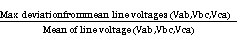(8)

Where, Vab, Vbc&Vca are line-to-line RMS voltages

The compatibility level of VUF, as recommended by IEEE is less than 2%. 

Energy loss in the power system can be computed by the following three methods:

Computer simulation method

Statistical method.

From the three methods, the Loss-Load factor method is extensively used to compute distribution system energy losses, which can be expressed as: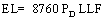(9)

Where, LLF is Loss-Load factor and PD is maximum power demand. The LLF in the above equation can be calculated from the following empirical formula , .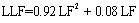(10)

Where, LF is the Load factor obtained from the following relation: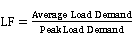(11)

The average and peak load demands are obtained from load measurements by using clamp on meter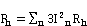(12)

where Rn, the ohmic resistance, depends on the harmonic frequency, nf1 and In stands for harmonic order currents starting from first order to nth order.

In general, the presence of harmonics (non linear loads) increases the losses in distribution transformers by 24% as that compared with losses in the presence of linear loads. [10, 11]

2. Research Methodology

This study makes use of measurement and simulation methods. All required distribution system data of study area data such as transformer MVA, %Z, %R, feeder length, feeder(cable) impedance/km, feeder resistance/km and feeder reactance/km that are necessary for simulation purpose are collected from name plates of equipment , interviewing substation engineers and referring to relevant documentations in the substation.

Periodic meter reading of already installed kWh and kVAr meters is also used for determination of average power factor. The meter readings are taken on hourly basis for ten (10) consecutive days.

A Clamp-on meter is used for measuring current and voltage and then to determine hourly power demand of each phase at the service transformer’s secondary sides. Load current measurements and line -to-line voltage measurements are carried out for ten consecutive days on hourly basis to include the stochastic load nature. After gathering of all necessary data of distribution system components, modeling is carried out by DigSILENT power factory soft wareand simulation work is carried out for balanced and unbalanced scenarios. The result of simulation work is compared against accepted IEEE & NEC standards.

3. Results and Discussions

The main purpose of load flow analysis is to determine the voltage magnitude and angle at bus bars, to determine the active and reactive power flows through the network components and to determine the power loss in the network components and entire system

(1)  Power Loss: Power loss refers to waste energy along the feeders as a result of current flow. Loss along distribution lines/cables and distribution transformers, is obtained as the difference of power entering the line/cable/transformer and leaving the lines/cables/transformer. Fig1, Fig2 & Fig3 show the percentage power losses of network components at maximum power transfer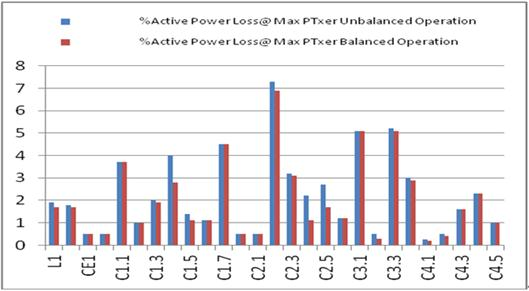Fig. 1. Percentage power Loss for Lines & Cables.

For the graph shown in Fig. 1, the vertical axis corresponds to percentage active power loss and horizontal axis refers to name of overhead lines & cables. As indicated on the graph, the percentage active power loss for four branch circuits violate the accepted standard of 1 to 4% when maximum power flows through them.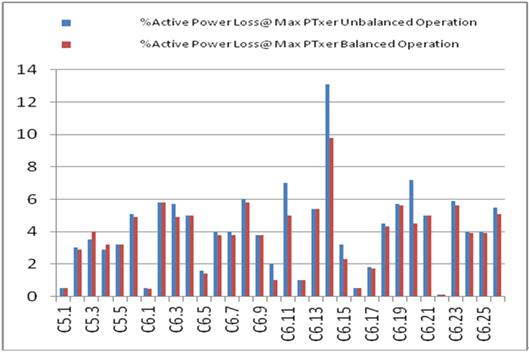Fig. 2. Percentage power Loss for Lines & Cables.

For the graph shown in Fig. 2, the vertical axis correspond to percentage active power loss and horizontal axis correspond to name (codes) of cables.

As indicated on the graph, the percentage active power loss for fourteen branch circuits violate the accepted standard of 1% to 4% when maximum power flows through them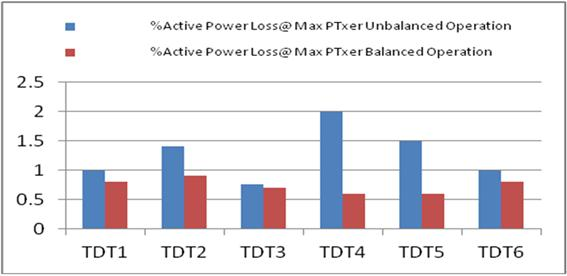Fig. 3. Percentage power Loss for transformers.

In the above graph, the values on the vertical axis correspond to percentage active power loss and the codes on the horizontal axis refer to name of distribution transformers. As indicated on the graph, the percentage power loss for distribution transformers is within the accepted limit of 1 to 4% 

(2)  Voltage Regulation

Voltage regulation result shows the variation in the magnitude of voltage at the load bus bars for wide range of system loading. For reliable operation of power system equipment, the voltage magnitude should remain within the NEC standard (0.95 to 1.05 P.U). The information shown in Fig. 4 shows the per unit (P.U) magnitude of voltage at various bus bars  for maximum network loading.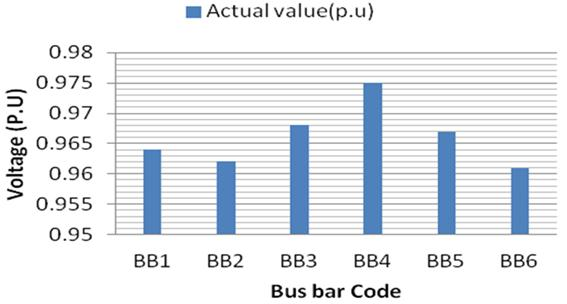Fig. 4. Voltage Regulations (P.U).

(3)  Power Factor Result

Power factor values tell us the ratio of active power flow to the apparent power flow in the system. The power factor values can be determined from energy meter recordings on hourly basis or on interval of 30 minutes. According to Ethiopian Electric Power Corporation (EEPCo) regulation, a power factor value of greater than 0.85 should be maintained in order to avoid penalty charge. The information shown in Fig5 indicates the average power factor values measured at the distribution transformers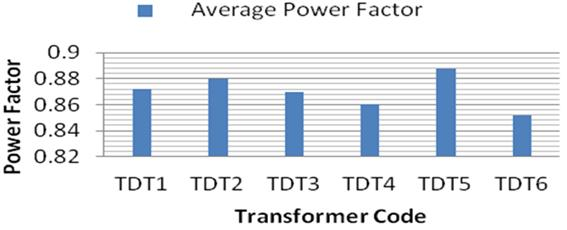Fig. 5. Power Factor data.

As indicated on the graph, except for one distribution transformer (TDT6), the values for other distribution transformers are within the EEPCo recommended value(>0.85)

(4)  Voltage Unbalance

Voltage unbalance factor refers to the deviation of phase voltages of the three phase system from their rated magnitude and phase difference. The vertical values in Fig6 represents percentage voltage unbalance factor.Fig. 6. Voltage Unbalance Factor.

As shown by the graph, the voltage unbalance factors are all within the IEEE Standard of less than 2%. However, the values at BB3 & BB6 are relatively larger as compared to others

4. Loss Minimization

Losses which occur in distribution system cost utilities a sizable amount of profit and hence need be reduced. Many techniques can be used to reduce the losses. some of them are done during an initial design and installation stage such as selection of efficient distribution transformer and its optimal location to reduce the length of distribution lines, selection less resistive conductor materials and use of as high voltage profiles as possible. Other techniques can be done on the existing system such as use of shunt capacitors , placement of harmonic filters, load balancing and demand side management. In this paper, loss minimization by shunt capacitors is used. As shown on the results section, percentage losses at maximum power transfer for 18 branch circuits are above the recommended value (1 to 4%). Moreover, the overall percentage loss in the distribution system is 7.4%, which is above the normal percentage loss in power distribution system of 3% to 6% .Therefore, individual shunt capacitor banks at the candidate branch circuits should be installed to bring the losses down to the accepted value. Network reconfiguration, as loss reduction method, is also recommended, however, it has a drawback of increasing the short circuit capacity of transformers and ratings of protective devices.Table1 shows capacitors size to be connected at the candidate bus bars to improve the power factor values. The capacitor banks are sized according to the equation given in 

Table 1. Shunt Capacitor Size for Textile Factory.

 Branch Name Present Power Factor Desired Power Factor Required capacitor  Bank Size (kVAr) C1.7 0.87 0.95 80 C2.2 0.87 0.95 150 C3.1 0.87 0.95 120 C3.3 0.87 0.95 60 C5.6 0.87 0.95 130 C6.2 0.88 0.95 50 C6.3 0.88 0.95 25 C6.4 0.88 0.95 25 C6.8 0.88 0.95 2.5 C6.11 0.88 0.95 2.5 C6.13 0.88 0.95 2.5 C6.14 0.88 0.95 10 C6.18 0.88 0.95 5 C6.19 0.88 0.95 10 C6.20 0.88 0.95 10 C6.21 0.88 0.95 2.5 C6.23 0.88 0.95 5 C6.26 0.88 0.95 25

Connection of these capacitor banks at the candidate branch circuits and simulating the distribution system decreases the total power loss from 413.5 kW to 376 kW (power loss saved is 37.5 kW). The annual energy loss saved is thus equal to 37.5Kw*8760Hrs=328.5 MWh. If energy tariff of 0.6088Birr/kWh for industrial consumer is considered, the loss of 199,991 Birr is saved.

5. Conclusions

Study of Reactive power flow, power loss and voltage quality problems of distribution system was made in this paper. Voltage regulation and voltage unbalance factors at the case study area are within the recommended limits. Percentage power losses at maximum power transfer for some branch circuits in the case study area are above the recommended value (1 to 4%). Moreover, the overall percentage loss in the system is 7.4% of the total power input, which is greater than the recommended loss percentage of 3 to 6% in distribution system. Individual reactive power compensation at these branch circuits saves annual energy loss of 328.5 MWh.

References

1. Renewable energy and energy efficiency Partnership,"Energy efficient systems in Development of BuildingRegulations and Guidelines to Achieve Energy Efficiencyin Bangalore City".
2. Vic Gosbell, "Voltage unbalance and Its Effects", 2002.
3. Betrianisdan Randite, Herawan, "Analysis of Technical Energy Losses by using Simulation" Makara, Teknologi,Vol.10.no.1, April 2006:11-17.
4. M.W Gustafson",Demand, Energy and Marginal Electrical System Losses", IEEE 83 WM 176-5, 1982.
5. J.Schlabbach, D.Blume and T.Stephanblome, "Voltage Quality in ElectricalPower systems", 2000.
6. DIgSILENT Power Factory User Manual:http:/www.digsilent.de/software/DIgSILENT Power Factory.
7. Latest shunt capacitor price list: www.nepsi.com.
8. IEEE Recommended Practices and Requirements for Harmonic Control in Electrical Power Systems, IEEE Standard 519-1992, IEEE Publications, New York, USA:The Institute of Electrical and Electronics Engineers, Inc,1993.
9. S.B.Sadati, H.Yousefi, B.Darvishi, and A.Tahani, "Comparison of Distribution Transformer Losses and Capacity under Linear and Harmonic Loads," presented at the 2nd IEEE International Conference on Power and Energy (PECon 08), December 1-3, 2008, Johor Baharu, Malaysia.
10. Benedict,E.; Collins,T;Gotham,D.; etal, "Losses inElectric Power Systems"(1992).
11. T.Gonen, Electric Power Distribution system Engineering, 1986.

 Contents 1. 2. 3. 4. 5.
Article ToolsAbstractPDF(1571K)